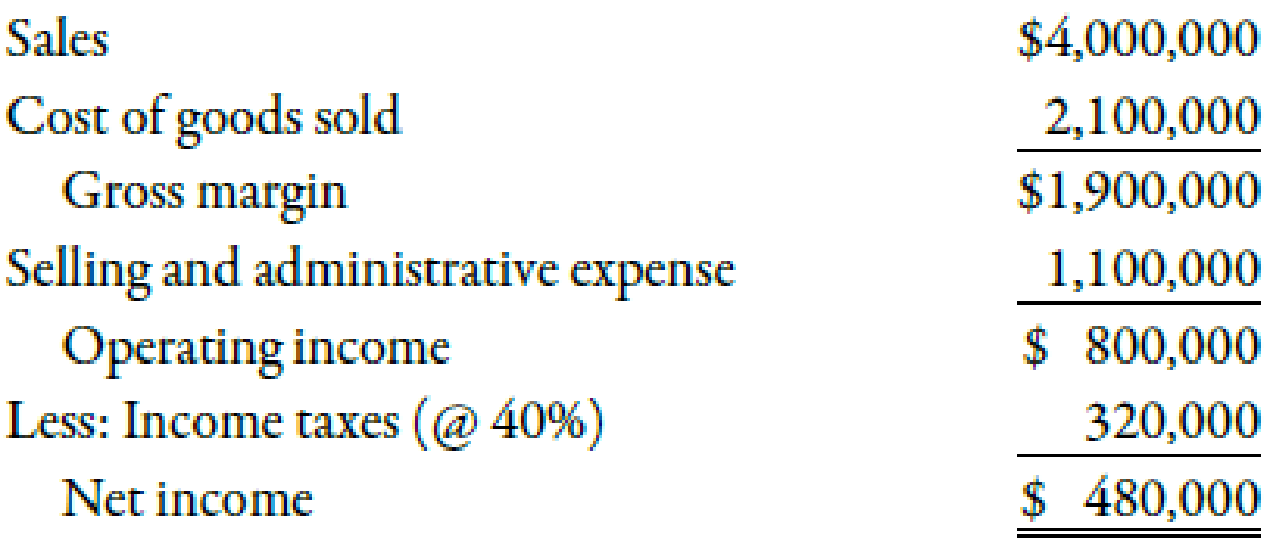Chapter 11, Problem 20BEB### Managerial Accounting: The Corners...

7th Edition
Maryanne M. Mowen + 2 others
ISBN: 9781337115773

#### Solutions

Chapter
Section### Managerial Accounting: The Corners...

7th Edition
Maryanne M. Mowen + 2 others
ISBN: 9781337115773
Textbook Problem
35 views

# Use the following information for Brief Exercises 11-19 through 11-21:Barnard Manufacturing earned operating income last year as shown in the following income statement:At the beginning of the year, the value of operating assets was $2,700,000. At the end of the year, the value of operating assets was$2,300,000.Brief Exercise 11-20 Calculating Residual IncomeRefer to the information for Barnard Manufacturing on the previous page. Barnard requires a minimum rate of return of 15%.Required:Calculate (1) average operating assets and (2) residual income.

(1)

To determine

Compute average operating assets.

Explanation

Average Operating Assets:

Average operating assets can be determined by adding the beginning assets and ending assets and dividing the result by 2.

Use the following formula to calculate average operating assets:

Average operating assets=(Beginning assets+Ending assets)2

Substitute $2,700,000 for beginning assets and$2,300,000 for ending assets in the above formula

(2)

To determine

Compute residual income.

### Still sussing out bartleby?

Check out a sample textbook solution.

See a sample solution

#### The Solution to Your Study Problems

Bartleby provides explanations to thousands of textbook problems written by our experts, many with advanced degrees!

Get Started

#### Find more solutions based on key concepts